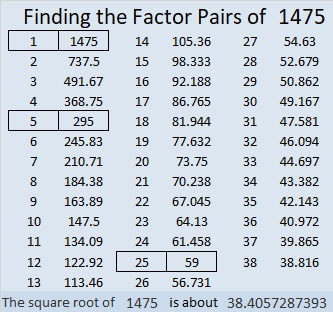# 1475 The Logic Needed to Solve This Mystery

Contents

### Today’s Puzzle:

Solving this puzzle will be as easy as 1-2-3 if you begin by asking yourself which clues must use the 1’s, the 2’s, and the 3’s, but not necessarily in that order. At the same time, you can eliminate two common factors of 48 and 72, and finishing the puzzle should then become fairly routine.### Factors of 1475:

• 1475 is a composite number.
• Prime factorization: 1475 = 5 × 5 × 59, which can be written 1475 = 5² × 59
• 1475 has at least one exponent greater than 1 in its prime factorization so √1475 can be simplified. Taking the factor pair from the factor pair table below with the largest square number factor, we get √1475 = (√25)(√59) = 5√59
• The exponents in the prime factorization are 2 and 1. Adding one to each exponent and multiplying we get (2 + 1)(1 + 1) = 3 × 2 = 6. Therefore 1475 has exactly 6 factors.
• The factors of 1475 are outlined with their factor pair partners in the graphic below.### Another Fact about the Number 1475:

1475 is the hypotenuse of two Pythagorean triples:
413-1416-1475 which is (7-24-25) times 59.
885-1180-1475 which is (3-4-5) times 295.

This site uses Akismet to reduce spam. Learn how your comment data is processed.